Java Swing GUI

# How to draw lines, rectangles, and circles in JFrame

In this tutorial, we are going to see how to draw lines, rectangles and circles in JFrame. Java offers us an easy way to draw graphics using Graphics class in AWT package which allows us to draw primitive geometric types like lines, circles, etc… This tutorial explains the different functions of Graphics class used to draw shapes.

###### Draw a line

Graphics class provides the Graphics.drawline(int x1, int y1, int x2, int y2) method to draw a line on the screen. While x1 is the x-coordinate of the first point in the line and y1 is the y-coordinate of the first point in the line. Similarly, x2 and y2 are the coordinates of the second point in the line.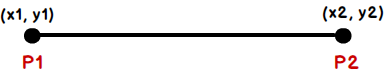Here is the program that draws a line.

```import java.awt.Graphics;
import javax.swing.*;

public class DrawMyLine extends JPanel
{
public void paint(Graphics g){

g.drawLine(20, 20, 200, 180);
}

public static void main(String[] args){
JFrame f = new JFrame("Draw a line");
f.setSize(250, 250);
f.setVisible(true);
f.setResizable(false);
f.setDefaultCloseOperation(JFrame.EXIT_ON_CLOSE);
}
}```

Output: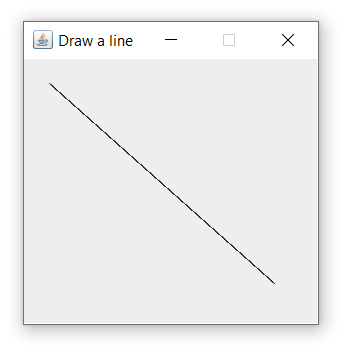###### Draw a circle

You can draw a circle and oval using the Graphics.drawOval(int x, int y, int width, int height) method. This function performs both functions. ‘x’ and ‘y’ are the starting point on the screen, and ‘width’ and ‘height’ are the parameters to set the width and height of the oval or circle. For the circle, set the same width and height.Here is the program that draws a circle on the screen.

```import java.awt.Graphics;
import javax.swing.*;

public class DrawMyCercle extends JPanel
{
public void paint(Graphics g){

g.drawOval(50, 40, 150, 150);
}

public static void main(String[] args){
JFrame f = new JFrame("Draw a circle");
f.setSize(250, 250);
f.setVisible(true);
f.setResizable(false);
f.setDefaultCloseOperation(JFrame.EXIT_ON_CLOSE);
}
}```

Output: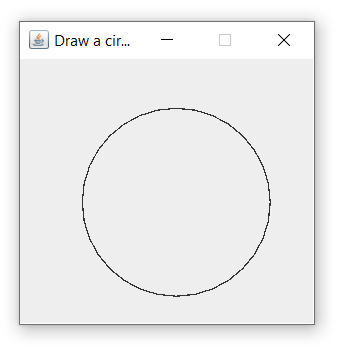###### Draw a rectangle

Graphics class provides the Graphics.drawRect(int x, int y, int width, int height) method for drawing a rectangle or square. The first two parameters specify the starting point and the last two parameters specify the width and height of the rectangle or square. The width and height of the square must be identical.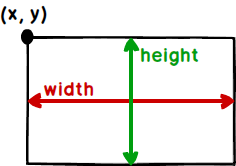Here is the program that draws a rectangle on the screen.

```import java.awt.Graphics;
import javax.swing.*;

public class DrawMyRect extends JPanel
{
public void paint(Graphics g){

g.drawRect(50, 35, 150, 150);
}

public static void main(String[] args){
JFrame f = new JFrame("Draw a rectangle");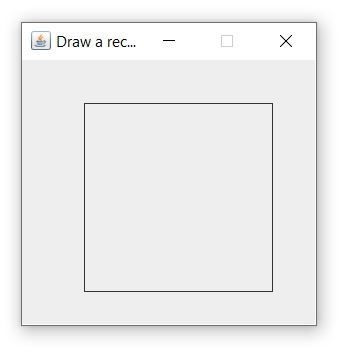MCQPractice competitive and technical Multiple Choice Questions and Answers (MCQs) with simple and logical explanations to prepare for tests and interviews.Read More